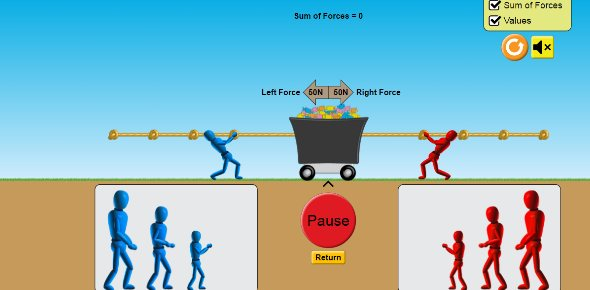# Force And Motion Vocabulary Quiz (Sol 4.2)

7 Questions | Total Attempts: 139SettingsThis quiz is designed to see how well you know the vocabulary in the unit.

• 1.
What is potential energy?
• A.

Energy of motion

• B.

Energy an object has because of its position, shape or condition

• 2.
What is kinetic energy?
• A.

Energy of motion

• B.

Energy an object has because of its position, shape or condition

• 3.
What is mass?
• A.

How fast something is going

• B.

Them amount of 'stuff' in an object

• 4.
What is velocity?
• A.

Holding a ball high so it will fall faster

• B.

How fast and in what direction an object is going

• 5.
What is gravity?
• A.

How much something weighs

• B.

A force that pulls all objects down

• 6.
What is weight?
• A.

Measure of the force of gravity on an object

• B.

The amoutn of 'stuff' in something

• 7.
What is friction?
• A.

A force that keeps objects that are touching each other from sliding past each other easily

• B.

A push or pull

Related TopicsBack to top